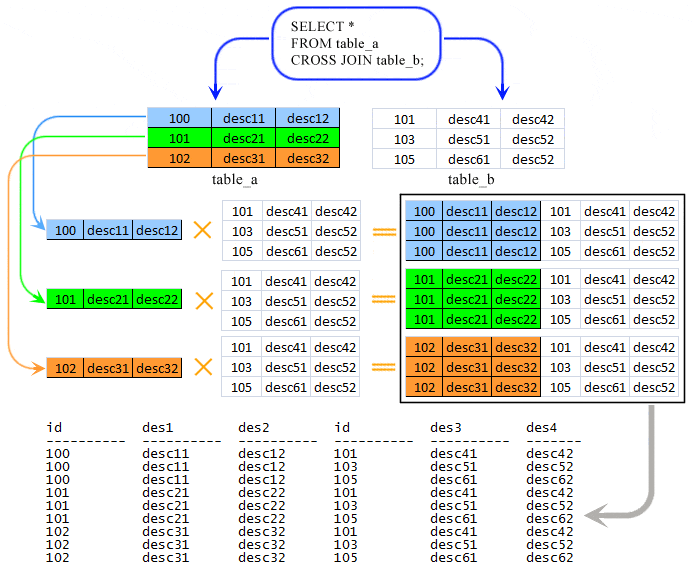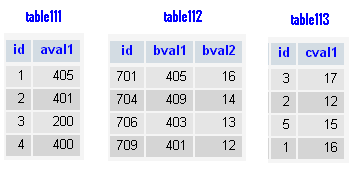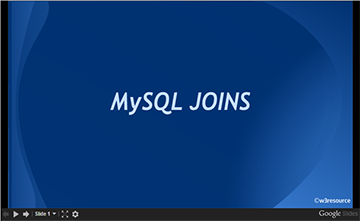# MySQL CROSS JOIN

## Cross Join

In MySQL, the CROSS JOIN produced a result set which is the product of rows of two associated tables when no WHERE clause is used with CROSS JOIN.

In this join, the result set appeared by multiplying each row of the first table with all rows in the second table if no condition introduced with CROSS JOIN.

This kind of result is called as Cartesian Product.

In MySQL, the CROSS JOIN behaves like JOIN and INNER JOIN of without using any condition.

In standard SQL the difference between INNER JOIN and CROSS JOIN is ON clause can be used with INNER JOIN on the other hand ON clause can't be used with CROSS JOIN.

Pictorial presentation of MySQL CROSS JOIN :MySQL CROSS JOIN Syntax:

MySQL supports the following JOIN syntaxes for the table_references (A table reference is also known as a join expression.) part of SELECT statements and multiple-table UPDATE and DELETE statements:

```table_references:
escaped_table_reference [, escaped_table_reference] ...

escaped_table_reference:
table_reference
| { OJ table_reference }

table_reference:
table_factor
| join_table

table_factor:
tbl_name [PARTITION (partition_names)]
[[AS] alias] [index_hint_list]
| table_subquery [AS] alias
| ( table_references )

join_table:
table_reference [INNER | CROSS] JOIN table_factor [join_condition]
| table_reference STRAIGHT_JOIN table_factor
| table_reference STRAIGHT_JOIN table_factor ON conditional_expr
| table_reference {LEFT|RIGHT} [OUTER] JOIN table_reference join_condition
| table_reference NATURAL [{LEFT|RIGHT} [OUTER]] JOIN table_factor

join_condition:
ON conditional_expr
| USING (column_list)

index_hint_list:
index_hint [, index_hint] ...

index_hint:
USE {INDEX|KEY}
[FOR {JOIN|ORDER BY|GROUP BY}] ([index_list])
| IGNORE {INDEX|KEY}
[FOR {JOIN|ORDER BY|GROUP BY}] (index_list)
| FORCE {INDEX|KEY}
[FOR {JOIN|ORDER BY|GROUP BY}] (index_list)

index_list:
index_name [, index_name] ...```

Example: MySQL CROSS JOIN

In the following example, a Cartesian Product has retrieved.

Code:

``````SELECT table112.id,table112.bval1,table112.bval2,
table111.id,table111.aval1
FROM table112
CROSS JOIN table111;
```
```

Sample tables:Sample Output:

```+------+-------+-------+------+-------+
| id   | bval1 | bval2 | id   | aval1 |
+------+-------+-------+------+-------+
|  701 |   405 |    16 |    1 |   405 |
|  704 |   409 |    14 |    1 |   405 |
|  706 |   403 |    13 |    1 |   405 |
|  709 |   401 |    12 |    1 |   405 |
|  701 |   405 |    16 |    2 |   401 |
|  704 |   409 |    14 |    2 |   401 |
|  706 |   403 |    13 |    2 |   401 |
|  709 |   401 |    12 |    2 |   401 |
|  701 |   405 |    16 |    3 |   200 |
|  704 |   409 |    14 |    3 |   200 |
|  706 |   403 |    13 |    3 |   200 |
|  709 |   401 |    12 |    3 |   200 |
|  701 |   405 |    16 |    4 |   400 |
|  704 |   409 |    14 |    4 |   400 |
|  706 |   403 |    13 |    4 |   400 |
|  709 |   401 |    12 |    4 |   400 |
+------+-------+-------+------+-------+
16 rows in set (0.05 sec)```

Example: MySQL CROSS JOIN with LEFT JOIN

In the following example, at first cross join between table112 and table133 have completed then executes the left join according to the specified condition.

Code:

``````SELECT *
FROM table111
LEFT JOIN(table112 CROSS JOIN table113)
ON table111.id=table113.id;
```
```

Sample Output:

```+------+-------+------+-------+-------+------+-------+
| id   | aval1 | id   | bval1 | bval2 | id   | cval1 |
+------+-------+------+-------+-------+------+-------+
|    1 |   405 |  701 |   405 |    16 |    1 |    16 |
|    1 |   405 |  704 |   409 |    14 |    1 |    16 |
|    1 |   405 |  706 |   403 |    13 |    1 |    16 |
|    1 |   405 |  709 |   401 |    12 |    1 |    16 |
|    2 |   401 |  701 |   405 |    16 |    2 |    12 |
|    2 |   401 |  704 |   409 |    14 |    2 |    12 |
|    2 |   401 |  706 |   403 |    13 |    2 |    12 |
|    2 |   401 |  709 |   401 |    12 |    2 |    12 |
|    3 |   200 |  701 |   405 |    16 |    3 |    17 |
|    3 |   200 |  704 |   409 |    14 |    3 |    17 |
|    3 |   200 |  706 |   403 |    13 |    3 |    17 |
|    3 |   200 |  709 |   401 |    12 |    3 |    17 |
|    4 |   400 | NULL |  NULL |  NULL | NULL |  NULL |
+------+-------+------+-------+-------+------+-------+
13 rows in set (0.00 sec)
```

Example: MySQL CROSS JOIN with WHERE clause

In the following example, CROSS JOIN have been executed with WHERE clause and it is similar to the INNER JOIN with ON clause.

Code:

``````SELECT table111.*,table113.*
FROM table111
CROSS JOIN table113
WHERE table111.id=table113.id;
```
```

Sample Output:

```mysql> select table111.*,table113.*
-> from table111
-> cross join table113
-> where table111.id=table113.id;
+------+-------+------+-------+
| id   | aval1 | id   | cval1 |
+------+-------+------+-------+
|    3 |   200 |    3 |    17 |
|    2 |   401 |    2 |    12 |
|    1 |   405 |    1 |    16 |
+------+-------+------+-------+
3 rows in set (0.05 sec)
```

Key points to remember

Click on the following to get the slides presentation -CROSS JOINS: SQL and other Relational Databases

Previous: STRAIGHT_JOIN
Next: NATURAL JOIN

﻿